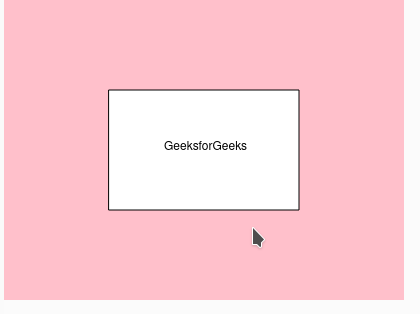GFG App
Open AppBrowser
Continue

p5.Geometry() method is used to represent 3d objects. It is returned by the loadModel() function and also used internally by the 3d primitive drawing functions.

This function requires p5.dom library. So add the following line in the head section of the index.html file.

## Javascript

 ``

Syntax:

`new p5.Geometry([detailX], [detailY], [callback])`

Parameters: detailX and detailY takes the number of vertices on a horizontal surface, callback takes a function to call upon object instantiation.

Available methods in p5.Geometry Class:

Example:

## Javascript

 `function` `setup() {  ` `  `  `    ``// Create Canvas of given size  ` `    ``var` `cvs = createCanvas(400, 300); ` `} ` `   `  `function` `draw() { ` `     `  `  ``// Set the background color ` `  ``background(``'pink'``);  ` `   `  `  ``// Creating rectangle at center of canvas ` `  ``rectMode(CENTER); ` `   `  `  ``// Initializing a rect geometry  ` `  ``geo = ``new` `p5.Geometry( ` `    ``rect(200,150,190,120) ` `  ``); ` `   `  `  ``// Adding text to the geometry figure ` `  ``text(``'GeeksforGeeks'``, 160, 150); ` `}`

• In new p5.Geometry( rect(200,150,190,120)), 200 is used to specify the x-axis, 150 for the y-axis and 190 is the width of the rectangle and, 120 is the height of the rectangle.
• Similarly, in text, 160 is the x-axis position and 150 is the y-axis position with respect to the canvas screen.

Output:Reference : https://p5js.org/reference/#/p5.Geometry

My Personal Notes arrow_drop_up